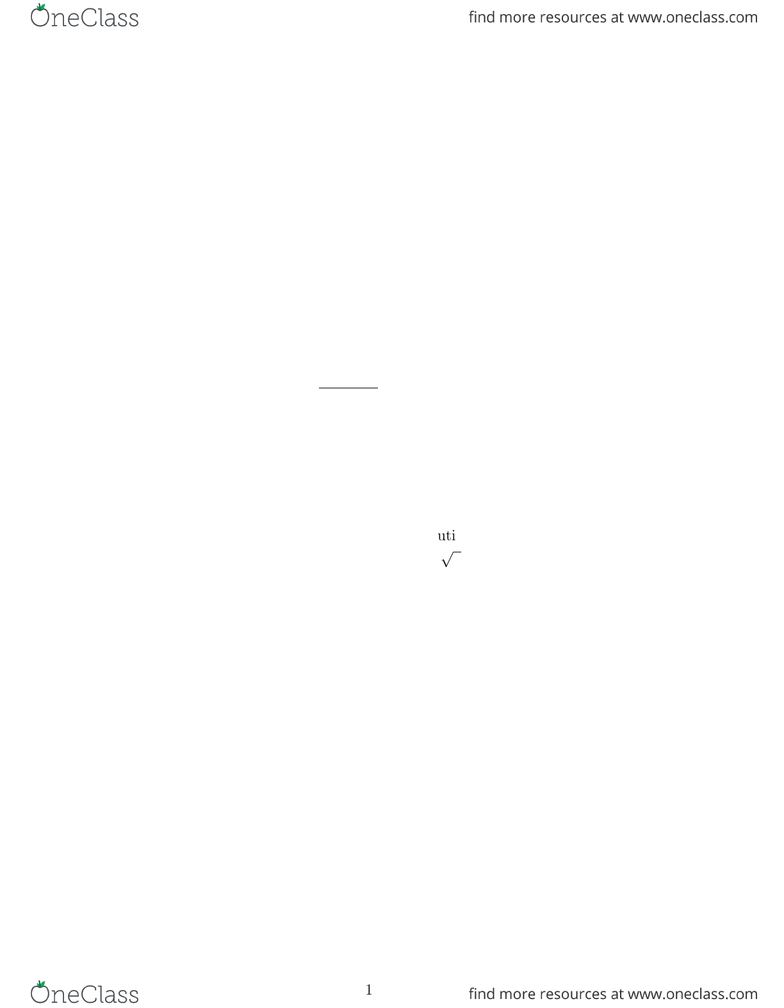Class Notes (1,000,000)
CA (620,000)
Carleton (20,000)
MATH (500)
MATH 1004 (100)
Lecture 3

MATH 1004 Lecture Notes - Lecture 3: Thai Baht

Department
Mathematics
Course Code
MATH 1004
Professor
Glenn Mc Rae
Lecture
3

Page:
of 2Week # 12 MATH1004
8.3 The Volume of a Solid of Revolution
The volume of a right circular cylinder is
Volume of Cylinder= π(radius)2(height) = πr2h.
We can derive the volume of a cylinder with a cylindrical hole, and hole is a cylinder
in its own. Vhole, of the remaining solid which is a shell, is given by
Vhole = 2π(average radius)(width of wall)(height) (1)
where
2wall width = rout rin
Examples:
1. Find the volume of the cylindrical solid (of revolution) obtained by rotating the
vertical line segment whose ends are at (x, x2) and (x, 1), and whose width is deﬁned
by the symbol dx, about the yaxis.
2. Find the volume of the cylindrical solid of revolution obtained by rotating the hor-
izontal line segment whose ends are at (0, y) and (y, y) and whose height is deﬁned
by the symbol dy, about the yaxis, 0 < y < 1.
3. Find the volume of the cylindrical solid of revolution obtained by rotating the ver-
tical line segment whose ends are at (x, 0) and (x, 2xx2) and whose width is deﬁned
by the symbol dx, about the yaxis. 0 < x < 2
4. Find the volume of the cylindrical solid of revolution obtained by rotating the
vertical line segment whose ends are at (x, 0) and (x, 2xx2) and whose width is
deﬁned by the symbol dx, about the xaxis.
Finding the Volume of a Solid of Revolution
1. Sketch the region
2. Decide on a typical slice The rule of thumb here is just lie the one for areas in
the previous section: For rotation about the either the xaxisor yaxis.
iIf it easier to describe the region with function of x, use a vertical slice, otherwise
ii Write all the expressions as functions of y(by ﬁnding the inverse functions) and use
a horizontal slice.
1
3. Find the volume of the slice, dV Use (1).
4. Find the limits f integration These are obtained by ﬁnding the extremities of
the region.
5. Write down the deﬁnite integral for the volume drop all terms containing
the square of either dx or dy from the expression for dV .
6. Evaluate the deﬁnite integral
Examples:
1. Find the volume of the solid of revolution obtained by rotating the region bounded
by the curves y=x2, the yaxis and the line y= 1 about the yaxis.
2. Find the volume of the cylindrical solid of revolution obtained by rotating the region
bounded by the curves y= 2xx2and y= 0 about yaxis.
3. Find the volume of the cylindrical solid of revolution obtained by rotating the region
bounded by the curves y= 2xx2and y= 0 about xaxis.
2